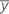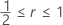# Methods and formulas for Outlier Test

Select the method or formula of your choice.

## Dixon's test statistics

Dixon's test determines whether the most extreme value in a sample is an outlier. Dixon's test includes a choice of test statistics that overcome the potential masking effects of other extreme values in the sample. Dixon's test statistic is denoted by rij , where the subscripts i and j indicate the following:
• i indicates the number of extreme values on the same side (lower or upper) of the data as the suspected outlier. i = 1 or 2.
• j indicates the number of extreme values on the opposite side of the data. j = 0, 1, or 2.

For example, if the suspected outlier is the smallest value in the sample, but the sample also includes two unusually large values, then r12 is the appropriate test statistic. The test statistic r10 , (also called Dixon's Q), is appropriate when the sample includes only one extreme value.

Critical values for Dixon's test statistics are tabulated in Rorabacher (1991).

### One-sided test statistics

The formula for the one-sided test depends on whether you test the smallest value, yi , or the largest value, yn. To test whether yi , is the outlier, use the following formula:
To test whether yn , is the outlier, use the following formula:

### Two-sided test statistics

We define the two-sided test statistic as King (1953) defines the two-sided test statistic related to r10. The two-sided test statistic is given by:

### Notation

TermDescription
rijDixon's test statistic (i = 1, 2; j = 0, 1, 2)
yithe ith smallest value in the sample
nthe number of observations in the sample

### References

• D.B. Rorabacher (1991). "Statistical Treatment for Rejection of Deviant Values: Critical Values of Dixon Q Parameter and Related Subrange Ratios at the 95 percent Confidence Level," Analytic Chemistry, 83, 2, 139-146.
• E.P. King (1953). "On Some Procedures for the Rejection of Suspected Data," Journal of the American Statistical Association, Vol. 48, No. 263, 531-533.

## Grubbs' test statistic

### Formula for the one-sided statistic

If you test whether the smallest data value is an outlier, then the test statistic G is given by:
If you test whether the largest data value is an outlier, then G is given by:

### Formula for the two-sided statistic

For a two-sided hypothesis, G is given by:

### Notation

TermDescriptionthe sample mean
yithe ith smallest value in the sample
sthe standard deviation of the sample
nthe number of observations in the sample

## P-values for Dixon's test statistics

Assuming that the data are normally distributed, the Dixon statistics have the same distribution whether you test the smallest value or the largest value. So, without any loss of generality, we may focus on the statistics for detecting outliers in the high end of the data, namely:

### Cumulative distribution function for the test statistic

According to Dixon (1951) and McBane (2006), the probability density functions of the distribution of the test statistics rij may be written as:
where C is the normalizing factor specified by:
and the Jacobian J(x,v,r) is specified by:
Using the transformation where t = (1 + r2 ) v2 / 2 and u2 = 3x2 / 2, the density function may be rewritten as:

Minitab evaluates the inner integral using a 30-point Gauss-Laguerre quadrature. Minitab evaluates the outer integral using a 30-point Gauss-Hermite quadrature.

The cumulative distribution functions for the family of test statistics are specified by:

Similar to McBane (2006), Minitab calculates Fij(r) using a 16-point Gauss-Legendre quadrature method.

### P-value for one-sided test

For any pair of subscripts (i, j), the p-value for the observed one-sided statistic, r, is specified by:

### P-value for one-sided test

Using King's (1953) result, for any pair of subscripts (i, j), the p-value for the observed two-sided statistic, r, is specified by:

Also, King observes that the above approximation becomes an equality for.

### Notation

TermDescription
rijthe Dixon test statistic where i = 1, 2; j = 0, 1, 2
yithe ith smallest value in the sample
nthe number of observations in the sample

### References

W.J. Dixon (1951). "Ratios Involving Extreme Values," Annals of Mathematical Statistics, 22(1), 68-78.

E.P. King (1953). "On Some Procedures for the Rejection of Suspected Data," Journal of the American Statistical Association, Vol. 48, No. 263, pages 531-533.

G.C. McBane (2006). "Programs to Compute Distribution Functions and Critical Values for Extreme Value Ratios for Outlier Detection," Journal of Statistical Software, Vol. 16, No. 3, pages 1-9.

## P-values for Grubbs' test statistic

### Formula for a one-sided test

The p-value for a one-sided test is:

### Formula for a two-sided test

The p-value for the two-sided test is:

### Exact versus approximate p-values

If the following is true, then the p-value is exact.

If not, the calculated p-value represents an upper bound for the exact p-value. However, the upper bound is a very good approximation of the exact p-value.

### Notation

TermDescription
GGrubbs' test statistic
nthe number of observations in the sample
Ta random variable distributed as a t-distribution with n – 2 degrees of freedom
By using this site you agree to the use of cookies for analytics and personalized content.  Read our policy